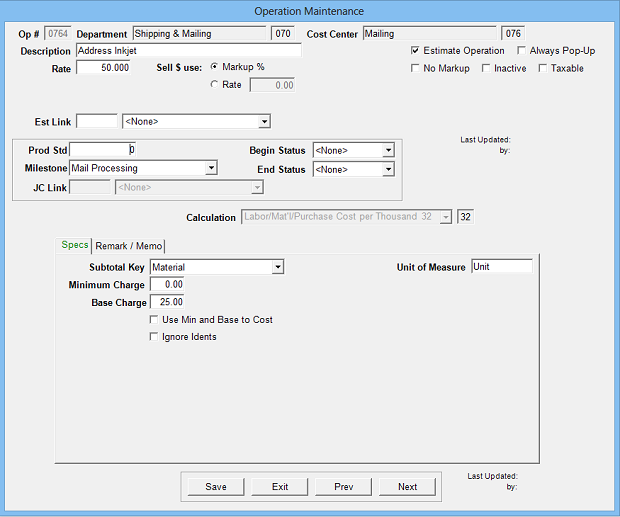Estimates the cost of a labor, material, or outside purchase by the cost per thousand.

Operation Setup Requirements:
1. Description
2. Rate: Cost per thousand units
3. Sell \$ use: Markup % (recommended) or alternate sell rate
4. Calc:32
5. Sub Key: Labor, Material or Fixed Purchase
6. Minimum Charge: Option to define minimum dollar charge
7. Base Charge: Option to define base dollar charge
8. Use Min and Base to Cost: adds the Min & Base charges to the actual cost when posted to Job Control
9. Ignore Idents: Option to turn of ident tracking from formEstimate Specification Input Prompts:

1. Number Up
2. Idents
3. J% or Markup %
4. \$ Rate per Thousand
5. Remarks
6. MemoCalculation:

((Estimate Quantity / Number Out) x Idents)/ 1000 x \$ Rate + Base Charge = Cost \$

If Cost \$ less than Minimum Charge, then Cost \$ = Minimum Charge

Cost \$ x Markup % = Markup \$

Cost \$ + Markup \$ = Sell \$